{{announcement.body}}
{{announcement.title}}

# Forward and Back-Propagation Programming Technique/Steps to Train an Artificial Neural Net

DZone 's Guide to

# Forward and Back-Propagation Programming Technique/Steps to Train an Artificial Neural Net

### Forward and Back-Propagation programming technique/steps to train an Artificial Neural Net

· AI Zone ·
Free Resource

Comment (0)

Save
{{ articles.views | formatCount}} Views

This write-up is especially for those who want to try their hands at coding an Artificial Neural Net. How it is done mathematically doesn’t need an explanation from someone like me who is a programmer and not a scientist or a researcher. There are numerous training videos that you can go through and learn. I have gone through Prof Patrick Winston’s class as part of MIT OpenCourseWare and understood how the feed-forward and back-propagation technique works.

Through this article, I will explain the steps that you will need to follow to build a fully configurable ANN program (with N number of input features, N number of hidden layers, N number of neurons in each hidden layer, N number of output neurons). I would encourage to write your own custom program following the steps. As long as we adhere to the best practices of programming, test its efficiency, performance, we are good to go.

I did the same when I wrote my own program. I had the math with me and built the program one function at a time. The best way to know that your program is somewhat working is to pick an existing example/dataset from the internet and compare your program's output with that.

Take the following as an example. Input features = 3 (extra neuron is the Bias), Hidden Layers (5 Neurons, extra one is the Bias), 2 Output Neurons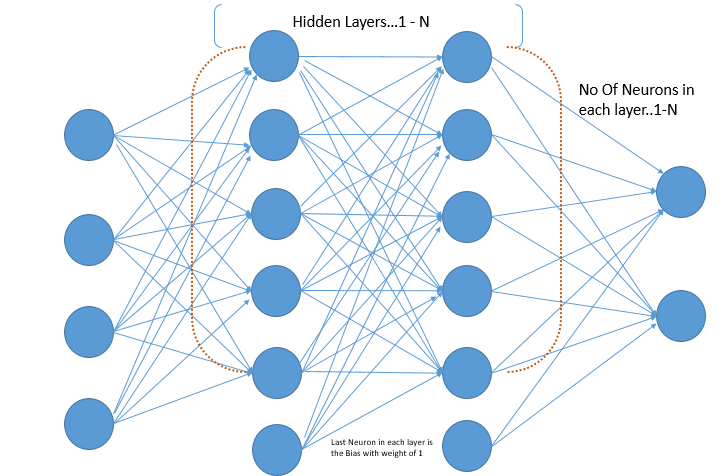It is very important that we first understand how the weight data structure works. Every Neuron in each layer will have a connection from every neuron from the preceding layer (including the Bias neuron). That would mean the following:

• Each neuron in Hidden Layer 1 will have 4 weights (1 from 3 input features and 1 extra for the Bias).
• Each neuron in Hidden Layer 2 and subsequent Hidden layers will have 6 weights (1 for those 5 neurons of the preceding layer and 1 extra for the Bias).
• The output neurons will each have 6 weights again (1 for those 5 neurons of the last hidden layer and 1 extra for the Bias).

The Weight Array will look like this, and I will explain in the next steps how to build this Weight Array.

Weight Array for this example with ‘Input features = 3 (1 extra neuron for the Bias), Hidden Layers (5 Neurons, extra one for the Bias), 2 Output Neurons will look something like this

Hidden layer 1 (Outer Array Size of 5 for those 5 neurons and each neuron with an array of 4 weights)

Plain Text

Hidden layer 2 (Outer Array Size of 5 for those 5 neurons and each neuron with an array of 6 weights)

Plain Text

Output layer (Outer Array Size of 2 for those 2 neurons and each neuron with an array of 6 weights)

Plain Text

## Programming Steps

### Step 1

Define a function to train the network. It is always advisable to start with training one sample and then extending it to your complete dataset. It is easier to debug, and what you will do for one sample will be applicable to all samples (running in a FOR loop the same steps for each row in the dataset)

--RUN for N Number of Iterations in a FOR Loop

-- For each row in the Input Array of Sample Data, do the following operations

-- forward_propagate(parameter 1, parameter 2, ….)

-- calculate the performance(Desired - Output)

-- backward propagate (parameter 1, parameter 2, …)

-- update weights(parameter 1, parameter 2, …)

### Step 2

Initiate the Weight Array as per the structure given above:

• You should first loop through the Number of Hidden Layers
1. If it is the first hidden layer
• Then run the FOR loop on the number of Input Features + 1, create random weights, and add to the weight array
• From the second hidden layer onwards, the FOR loop should run on the number of ‘Number of Neurons in the Hidden Layer + 1’, create random weights, and add to the weight array
• For the output layer, loop through the number of Output Neurons(outer loop).
1. The inner loop should be over the ‘Number of Neurons in the hidden layer + 1’, create random weights and add to the weight array

You will have to take care of `sub_arrays`, `main_arrays`, and Dictionary objects, while you are building the weighted network, as this is the most important initialization step

### Step 3

In the Forward Propagate, we will be trying to calculate the output by first multiplying each input by the corresponding weight of each neuron and then passing each neuron output through the Activation/Sigmoid function. We will do this for each neuron in each hidden layer, including the output layer.

The hidden layer data structure post-processing will look like this. Explanation on how to program the function is given below

Hidden Layer 1: 5 output for 5 neurons

Plain Text

Hidden Layer 2: 5 output for 5 neurons

Plain Text

Output Layer: 2 output for 2 neurons

Plain Text

The high-level programming steps are as follows:

• Loop though the Weight Network that you have built in step 2.
• For the first hidden layer:
1. Take each weight `sub_array`, multiply each of the first 3 weight elements out of 4 weights by each of the 3 input features, and leave the last weight element as that is for the Bias.
2. Now, take the last element weight `sub_array[-1]` and multiply by +1.
3. Pass the aggregate through the sigmoid `…1/(1+np.exp(-aggregate))`.
4. Add the output to the hidden layer output array. (It will look like the sample data above.)
5. Set the output as the new input and repeat the loop. (This is an input to the next hidden layer.)
• For the second hidden layer onwards, including the output layer, the process is the same
1. But, we will have more weights now as the number of neurons is more. If you scroll up, you will see that the Hidden layer output from Hidden layer 1 is of size 5, and the weight sub_array(of the weight network) of each neuron from hidden layer 2 is 6. So, 5 elements of the hidden layer will be multiplied by 5 weights of each neuron. (Each hidden layer output will be an input now and have a connection to every other neuron in that layer.) The last weight element will be multiplied by +1 (for the bias).
2. The Sigmoid function will be applied to the aggregate.

The final output that will come out of the Output Neuron layer will have an array size the same as the number of output neurons. (In this example that I am running, the size will be 2.)

### Step 4

This is the simplest of all the functions.

• We have the final output of those 2 output neurons, as given above [0.96415592452997234, 0.9223855095902298]]).
• Loop through these. In this example, the loop will run twice and subtract the desired value(d) we pre-defined in an output array with the above values(z). This may not be a pure subtraction, but we can do something like “Performance(P) = -(1/2)(d-z)**2”. This is only for mathematical convenience, as Prof Winston put it. The function makes the partial derivative w.r.t (z) simple and negates the negative sign as well. If you do a Partial Derivate of this performance function, you will get just (d-z), which is "Desired minus the Actual Output".

### Step 5

This is the most complex piece of the whole program, and you will have to take special care of how you are traversing the weight network, hidden layer outputs, and calculating the Delta_Error of each neuron as you are traversing backward

How the partial derivative is derived at and the calculation is explained in various courses. What we have to remember is the following chain rule and the same process of calculating can be extrapolated to N number of hidden layers and all Neurons.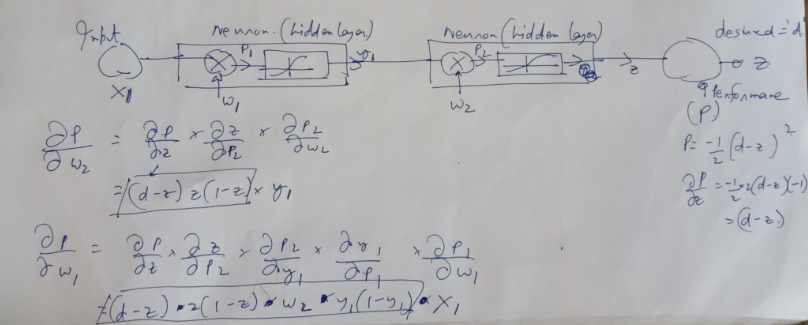The picture above shows the back propagation calculation for 1 neuron. Like the forward path, where every output from each neuron of each layer connects to every other neuron in the next layer, the delta error of each neuron in the next layer will have a contribution to the error calculation of each neuron of the preceding layer. I have explained the steps below. I am pasting the hidden layer output again below. This array will now be traversed in the reverse order

Hidden Layer 1: 5 output for 5 neurons

Plain Text

Hidden Layer 2: 5 output for 5 neurons

Plain Text

Output Layer: 2 output for 2 neurons

Plain Text

Loop through the weight network in the reverse order.

For i in reversed(range(len(weight_network))):

For the very first time, the if block below will be executed; then subsequent iterations will execute the else block until we reach the first hidden layer. With every iteration of the, reset the error array: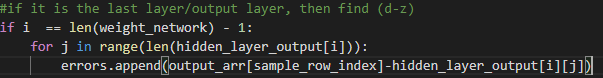Else: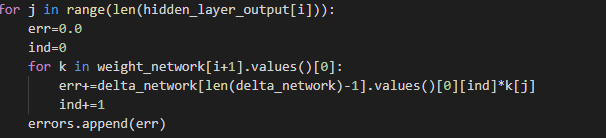Finally, outside the IF-ELSE, we will have to write the following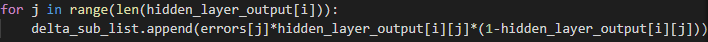The Delta network after the calculation will look like this:

Plain Text

The last output layer will have an array size of 2 as there are 2 output neurons, Hidden Layer 2 contribution to the error will have an array size of 5 as there are 5 neurons in the hidden layer 2, same for hidden layer 1

### Step 6

This is the step to update the weight network again. All we need in this step is the Weight Network, Hidden layer output array, and the Delta Error Network (all three, we have calculated above).

Before we proceed, we need to replace the last element of the Hidden layer output array, which is the output Layer output with the input elements (because if you look at the hand written calculation above, the partial derivative of the Performance w.r.t the first layer weight is X1-the input value)

Hidden Layer 1: 5 output for 5 neurons

Plain Text

Hidden Layer 2: 5 output for 5 neurons

Plain Text

Output Layer: 2 output for 2 neurons

Plain Text

The core logic for update weights is given in the snippet below

Python

Finally, to see if the program is behaving as expected, we can calculate the error value for every iteration (which is the performance function-Step #4). If the program is right, we will see a reduction in the error value at every iteration.

I ran the program with 200 iterations with a learning rate of 0.5 and got the following output.

Once you have the program ready, you can try different combinations of hidden layers, number of neurons in hidden layers, output neurons, learning rate, number of iterations to see how the program behavior changes, and how accurate your prediction gets.

Topics:
ANN, ai, ml, neural network, python, tutorial

Comment (0)

Save
{{ articles.views | formatCount}} Views

Opinions expressed by DZone contributors are their own.